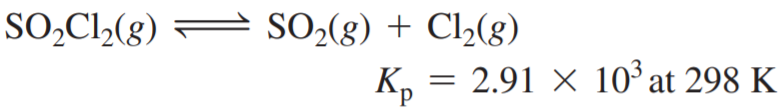×
Get Full Access to Chemistry: A Molecular Approach - 3 Edition - Chapter 14 - Problem 40e
Get Full Access to Chemistry: A Molecular Approach - 3 Edition - Chapter 14 - Problem 40e

×

# Consider the reaction: In a reaction at equilibrium, theISBN: 9780321809247 1

## Solution for problem 40E Chapter 14

Chemistry: A Molecular Approach | 3rd Edition

• Textbook Solutions
• 2901 Step-by-step solutions solved by professors and subject experts
• Get 24/7 help from StudySoup virtual teaching assistantsChemistry: A Molecular Approach | 3rd Edition

4 5 1 406 Reviews
12
2
Problem 40E

Consider the reaction:In a reaction at equilibrium, the partial pressure of SO2 is 137 torr and that of Cl2 is 285 torr. What is the partial pressure of SO2Cl2 in this mixture?

Step-by-Step Solution:

Solution 40EStep 1The reaction under consideration is represented as follows:The equilibrium constant. (Kp) of the reaction will be as follows:Here. is the partial pressure of is the partial pressure of is the partial pressure of SO2I2

Step 2 of 3

Step 3 of 3

##### ISBN: 9780321809247

The full step-by-step solution to problem: 40E from chapter: 14 was answered by , our top Chemistry solution expert on 02/22/17, 04:35PM. This full solution covers the following key subjects: torr, reaction, pressure, partial, mixture. This expansive textbook survival guide covers 82 chapters, and 9454 solutions. The answer to “?Consider the reaction: In a reaction at equilibrium, the partial pressure of SO2 is 137 torr and that of Cl2 is 285 torr. What is the partial pressure of SO2Cl2 in this mixture?” is broken down into a number of easy to follow steps, and 33 words. Chemistry: A Molecular Approach was written by and is associated to the ISBN: 9780321809247. Since the solution to 40E from 14 chapter was answered, more than 390 students have viewed the full step-by-step answer. This textbook survival guide was created for the textbook: Chemistry: A Molecular Approach, edition: 3.

Unlock Textbook Solution